# Calculus AB Review Course

Sale ends in
Teacher: William
Customers Who Have Viewed This Course: 3494
\$200.00
\$83.00

#### 1 Introduction 02:22

This video provides an overview of the course.

#### 2 Lesson 1: Logarithms 05:05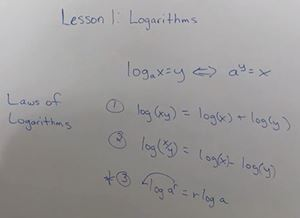This is part of the course’s limited pre-calculus material relevant to the Calculus AB exam. Here we look at exponential and logarithmic functions and their relationship to each other. This includes associated crucial cancellation equations and Laws of Logarithms which we need for this course.

#### 3 Lesson 1: Worksheet Review 06:40

This video goes through each of the practice problems from this lesson's worksheet. The answers are provided along with the methodology used to solve each problem, based on the lecture material. There is also a corresponding lesson answer key available, under the resource section, for quick reference.

#### 4 Lesson 2: Fundamental Graphs 06:27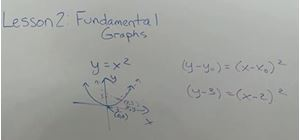This is part of the course’s limited pre-calculus material relevant to the Calculus AB exam. In this lesson we examine several fundamental functions and their corresponding graphs. We look at features of the graphs, as well as, how to generalize the functions and graphs to incorporate (x, y) translation. This material is of particular interest, as often we have to solve problems without a calculator and must rely upon knowledge of fundamental graphs (e.g., to assess an asymptote at infinity).

#### 5 Lesson 2: Worksheet Review 09:25

This video goes through each of the practice problems from this lesson's worksheet. The answers are provided along with the methodology used to solve each problem, based on the lecture material. There is also a corresponding lesson answer key available, under the resource section, for quick reference. We also discuss how to use a graphing calculator to find the intersection of two curves. This is an important skill needed to solve some of the AP exam’s calculator problems. For instance, we often need to do this to find the limits for a definite integral.

#### 6 Lesson 3: Trigonometry Part 1 07:02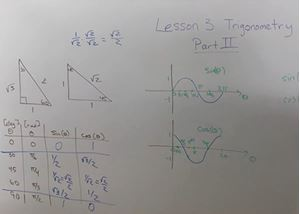This is the last lesson of the course’s limited pre-calculus material relevant to the Calculus AB exam. There is a lot of material packed in this lesson, as we look at key trigonometry items from various aspects. This includes trigonometry function relationship to the sides of a right triangle, Pythagorean’s Theorem, fundamental identities, value table construction, as well as, key trigonometric formulas and identities. We also discuss inverse / arc trigonometry functions.

#### 8 Lesson 3: Worksheet Review Part 1 04:53

This video goes through each of the practice problems from this lesson's worksheet. The answers are provided along with the methodology used to solve each problem, based on the lecture material. There is also a corresponding lesson answer key available, under the resource section, for quick reference.

#### 10 Lesson 4: Limits 10:27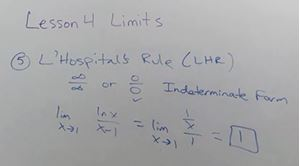Here we begin the Calculus portion of the course. We discuss the basic definition of a limit and how it relates to its one-sided limits. We look at how to leverage limits to identify vertical and horizontal asymptotes. Most of the lesson is focused on identifying and discussing how to solve five types of limit problems. This includes use of L’Hospital’s Rule for proper function indeterminate forms. The other four types of problems include direct, factoring, limits at infinity, and conjugate forms. We look at sample problems for each and related methodology for solving each type.

#### 11 Lesson 4: Worksheet Review 09:32

This video goes through each of the practice problems from this lesson's worksheet. The answers are provided along with the methodology used to solve each problem, based on the lecture material. There is also a corresponding lesson answer key available, under the resource section, for quick reference.

#### 12 Lesson 5: Continuity 05:31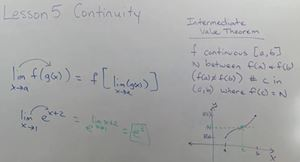Here we look at the definition of continuity and how it relates to the existence of the limit as x approaches a given value. We also look ahead briefly to derivatives and how to assess whether the derivative exists at specific points on a graph, such as at hard corners or cusps. We examine two key continuity theorems that give us a list of functions that are continuous by default and how we can move a limit within a corresponding equation containing these functions. Continuous functions include polynomials, rational, root, trigonometric, inverse trigonometric, exponential, and logarithmic functions. Lastly we examine the key Intermediate Value Theorem and discuss its utility. This is definitely something I would expect to see on the Calculus AB exam.

#### 13 Lesson 5: Worksheet Review 08:59

This video goes through each of the practice problems from this lesson's worksheet. The answers are provided along with the methodology used to solve each problem, based on the lecture material. There is also a corresponding lesson answer key available, under the resource section, for quick reference.

#### 14 Lesson 6: Limit Definition of a Derivative 07:40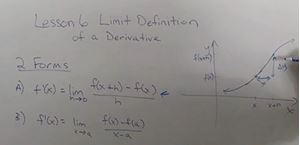We finish this section on this topic. As a natural transition to the subsequent derivative lesson, we examine the limit definition of a derivative and the two forms of the related equation. We look at several types of related problems including solving the formula directly, as well as, using the formula to identify an original function. Furthermore, we discuss the various cases where a function’s derivative does not exist such as cusps, discontinuities, and vertical tangents with sample graphs of each situation.

#### 15 Lesson 6: Worksheet Review 08:38

This video goes through each of the practice problems from this lesson's worksheet. The answers are provided along with the methodology used to solve each problem, based on the lecture material. There is also a corresponding lesson answer key available, under the resource section, for quick reference.

#### 16 Lesson 7: Derivatives 08:46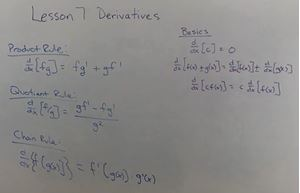In this lesson we discuss the most common functions and their associated derivatives. We review basic derivative formulas including taking the derivative of a constant, sum / difference of two functions, and a constant times a function. Furthermore, we review key power, product, quotient, and chain rules. We show how to use our developed derivative knowledge to solve, what I call, partial problems. This is where a table of sample x values and associated derivative values are given for one or more functions and we have to rely upon our formulas to develop a solution. We also discuss normal and tangent lines and how to develop formulas for these lines at a point, given the slope which we extract from the function’s derivative. Lastly, we discuss the most efficient formula for finding the derivative of an inverse function at a value.

#### 17 Lesson 7: Worksheet Review Part 1 09:55

This video goes through each of the practice problems from this lesson's worksheet. The answers are provided along with the methodology used to solve each problem, based on the lecture material. There is also a corresponding lesson answer key available, under the resource section, for quick reference.

#### 19 Lesson 8: Function First and Second Derivative Tests 07:57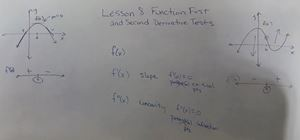Here we extend our derivative discussion to both first and second derivatives of a function and how to leverage to find key functional characteristics. We use the first derivative to examine a function’s slope and identify potential critical points (i.e., where the slope is zero). The second derivative gives us knowledge of the function’s concavity and potential inflection points (i.e., where the concavity is zero). We also discuss the Closed Interval Method to properly identify absolute minimum and maximum values overall a closed interval where both the end points and relative min / max values are examined together. Lastly, we show how to use the slope’s sign to identify intervals where a function is increasing or decreasing.

#### 20 Lesson 8: Worksheet Review 11:01

This video goes through each of the practice problems from this lesson's worksheet. The answers are provided along with the methodology used to solve each problem, based on the lecture material. There is also a corresponding lesson answer key available, under the resource section, for quick reference.

#### 21 Lesson 9: Implicit Differentiation 05:04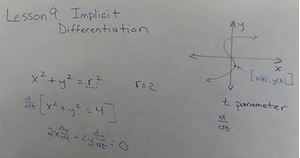We end the section by discussing implicit differentiation. Where before we took for granted a derivative being “with respect to” x, now we must consider cases where we are differentiating wrt other variables (e.g., parametric equations) and across different variables (e.g., d/dx of a function of y). We look at this form of differentiation in terms of derivative cancellation to help us solve problems, in general. We look at a sample parametric equation where we need to find the derivative of both x and y variables with respect to t, partly to prepare for related rates problems that we will be looking at and solving in a later lesson.

#### 22 Lesson 9: Worksheet Review Part 1 06:10

This video goes through each of the practice problems from this lesson's worksheet. The answers are provided along with the methodology used to solve each problem, based on the lecture material. There is also a corresponding lesson answer key available, under the resource section, for quick reference.

#### 24 Lesson 10: Integral Fundamentals 09:46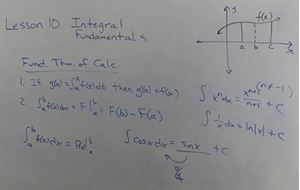After completing a comprehensive review of derivatives, we next turn our attention to another major Calculus topic, integration, as we begin this new section. Whereas a first derivative gives us information about a function’s slope, an integral provides a means for finding the precise area under a function’s curve. More specifically, an integral represents the net area under a curve, thus an integral with both positive and negative areas will give a net area result. There are two main types of integrals, indefinite integrals and definite integrals which require unique approaches to solve. We look at properties that apply to both, as well as, separate definite integral properties. We examine the Fundamental Theorem of Calculus (FTC) and the relationship between derivatives and integrals, including anti-derivatives. The latter we leverage to solve integrals.

#### 25 Lesson 10: Worksheet Review 10:36

This video goes through each of the practice problems from this lesson's worksheet. The answers are provided along with the methodology used to solve each problem, based on the lecture material. There is also a corresponding lesson answer key available, under the resource section, for quick reference. We also discuss how to use a graphing calculator to solve a definite integral. This is another key skill needed to solve some of the AP exam’s calculator problems. This allows us to solve complex integrals where we can’t easily find an anti-derivative solution. Furthermore, it is often more efficient to solve an integral this way, if the problem allows.

#### 26 Lesson 11: Riemann Sums and Graphical Integration 11:26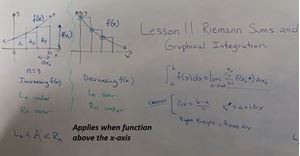When a discrete number of rectangles is used to cover the area under a curve, we form an integral approximation. This is called a Riemann sum. Typically we use either the left or right endpoints of each rectangle to establish the height. Other x values can be used, such as the midpoint, however the left and right points are helpful lower and upper bounds for the approximation. Furthermore we look at how to graphically integrate through use of simple half circle, rectangle, and triangle geometries where areas above the x-axis are positive and below the x-axis are negative to produce the precise net area under the overall curve. Lastly, we look at how to calculate an average value from a table of values to estimate the tangent line at a given x value.

#### 27 Lesson 11: Worksheet Review 11:27

This video goes through each of the practice problems from this lesson's worksheet. The answers are provided along with the methodology used to solve each problem, based on the lecture material. There is also a corresponding lesson answer key available, under the resource section, for quick reference.

#### 28 Lesson 12: Other Applications 06:13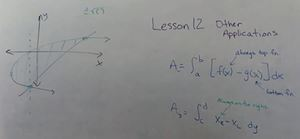In this lesson we examine other integral applications including finding the area between two curves and the average value of a general function. For area between two curve integrals, we look at when to evaluate the integral along either the x or y axis. Another aspect to these problems is establishing the limits of integration from the intersection of the two curves. Sometimes we can find these points by setting the functions equal to each other and then solving the resultant equation algebraically. For more complex problems, we need a calculator to assist us with finding the coordinates for key curve intersections. Lastly we look at finding the average value for any function with a straight-forward equation where we normalize the integral with one over the difference between the integral limits.

#### 29 Lesson 12: Worksheet Review 11:46

This video goes through each of the practice problems from this lesson's worksheet. The answers are provided along with the methodology used to solve each problem, based on the lecture material. There is also a corresponding lesson answer key available, under the resource section, for quick reference.

#### 30 Lesson 13: Substitution 09:02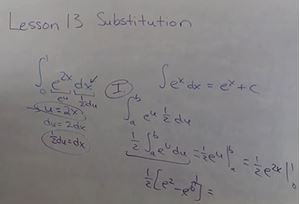Substitution allows us to reformat many integrations into a form that we can readily integrate. We look at two different methods for solving these problems. One, to back out the substitution variable (e.g., u) at the end, so that we can use the limits of the original integral. Two, to use our substitution equation to enable us to change the integral to an entirely different problem, where the new integral has limits directly related to our substitution variable. As part of using substitution, we not only need to develop a “u” substitution equation, but also a corresponding derivative equation (e.g., du). When properly scaled, this allows us to comprehensively replace, as required, the original variable and derivative with the proper “u” and “du” terms. We look at a typical substitution integral and walk through each step to solve it.

#### 31 Lesson 13: Worksheet Review 12:15

This video goes through each of the practice problems from this lesson's worksheet. The answers are provided along with the methodology used to solve each problem, based on the lecture material. There is also a corresponding lesson answer key available, under the resource section, for quick reference.

#### 32 Lesson 14: Abstract Problems 09:06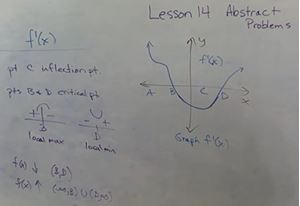There are many abstract problems on the AB exam which require knowledge of basic concepts. Several key insights are presented and discussed in the lecture to aid with solving these. One part of solving these problems is setting a frame of reference for the specific graph that is given. The characteristics that we need to extract from each problem will heavily depend on whether we are analyzing a raw function, first derivative, or second derivative graph. More so in this particular lesson, the worksheet problems and their review are a substantial element for learning this material.This is particularly important, as they are very relevant to the types of AP exam questions that you will need to later solve on your own. It is not just about getting the right answer here, but also about recognizing concepts and applying the appropriate technique(s).

#### 33 Lesson 14: Worksheet Review 13:56

This video goes through each of the practice problems from this lesson's worksheet. The answers are provided along with the methodology used to solve each problem, based on the lecture material. There is also a corresponding lesson answer key available, under the resource section, for quick reference.

#### 34 Lesson 15: Solving Problems Using Graphs 09:07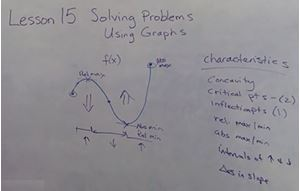In this lesson we continue our abstract problem discussion but focus more on easier graphical problems where we can extract a precise solution using the graph. We first review a sine-like function graph, over a closed interval, and discuss its various characteristics that can be leveraged. From the raw function graph, we can decipher absolute and relative minimum and maximum x values. We can also observe overall graph concavity and approximate location of inflection points. Relative min / max associated critical points can also be estimated. Lastly we can see intervals of function increase and decrease, as well as, visually see trends in slope as we move along the graph. Subsequently, we examine and discuss in detail raw function, 1st derivative, and 2nd derivative reference curves and corresponding characteristics that each provide us for a given underlying function. More specifically, we look at x-axis crossings, raw values, and curve slopes.

#### 35 Lesson 15: Worksheet Review 12:04

This video goes through each of the practice problems from this lesson's worksheet. The answers are provided along with the methodology used to solve each problem, based on the lecture material. There is also a corresponding lesson answer key available, under the resource section, for quick reference.

#### 36 Lesson 16: Particle Motion 11:31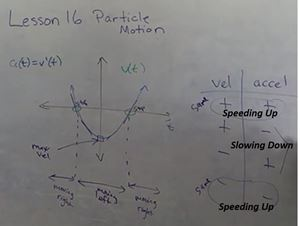Particle motion seems to be a significant focus on the AP exam. Typically for these problems, we are presented with a point-sized particle object moving along a line (e.g., the x-axis). We examine the relationship between position, velocity, and acceleration functions and how to develop each function from each other. This requires differentiation, in one direction, and integration when working backwards. Next we further discuss net displacement versus total distance traveled and how we need to use an absolute value operator to capture the total distance that a particle travels. A key feature needed here is to identify when the particle stops (i.e., v(t)=0), as these points indicate times when the particle likely changes direction. All of these concepts will be needed to solve the related AP exam questions. Again the worksheet problems are key and include a free response question on this topic.

#### 37 Lesson 16: Worksheet Review 14:53

This video goes through each of the practice problems from this lesson's worksheet. The answers are provided along with the methodology used to solve each problem, based on the lecture material. There is also a corresponding lesson answer key available, under the resource section, for quick reference.

#### 38 Lesson 17: Rates of Change and Related Rates 11:43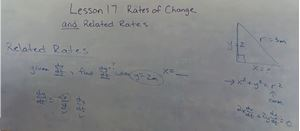There seems to be less of a focus on this material on the AP exam and partly why I decided to combine them into one lesson. Rates of change is really something that we can apply to just about any equation. In these problems, we basically are taking a derivative “with respect to” an independent variable. For example, in the case of a circle’s area, we can look at the rate of change of the area with respect to radius (i.e., dA/dr). In contrast, related rates is where we need to implicitly differentiate the equation wrt a third variable, typically time. A perfect example of this is the problem where you have a ladder sliding down a wall. In this case we use Pythagorean’s Theorem to give us a right triangle with the sides given by x and y and r for the hypotenuse. Differentiating wrt time produces associated rate terms for by x, dx/dt, and y, dy/dy.

#### 39 Lesson 17: Worksheet Review 11:49

This video goes through each of the practice problems from this lesson's worksheet. The answers are provided along with the methodology used to solve each problem, based on the lecture material. There is also a corresponding lesson answer key available, under the resource section, for quick reference.

#### 40 Lesson 18: Volume of a Solid 13:21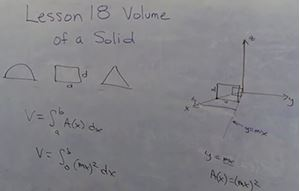In one type of problem, we use one or more curves that are rotated about either the x or y axis to outline the surface of the volume. This is partly formed with secondary constrains such as constant x or y values, or at the intersection of two curves. These curves are used to form the area of a cross-section in terms of x, when integrating along the x-axis, or in terms of y, when integrating along the y-axis. For the second type of problem, the constrained area between one or more curves forms the base of the solid, typically in the xy-plane. As we move along the x-axis, we erect a simple geometric shape whose base, perpendicular to the x-axis, varies. We use the specific shape given to calculate the associated cross-sectional area and integrate as before. The student will likely see a problem of the second type.

#### 41 Lesson 18: Worksheet Review 12:00

This video goes through each of the practice problems from this lesson's worksheet. The answers are provided along with the methodology used to solve each problem, based on the lecture material. There is also a corresponding lesson answer key available, under the resource section, for quick reference.

#### 42 Lesson 19: Differential Equations 10:22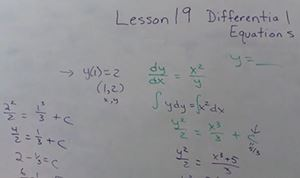This is an introductory lesson on differential equations (DE) and should be adequate for what you need to know on the Calculus AB exam. The simplest DE is, for example, the derivative of y “with respect to” (wrt) t equals a constant times y. Thus you see that a DE involves a function and its derivative in one equation. We use this particular DE in exponential growth and decay story problems. Next we look at separation of variables to solve harder DEs. Essentially, we isolate each variable and its associated derivative on either the left or right side of the equation and then integrate both sides to produce a y(t) solution equation. Lastly, we look at how to leverage simple DEs to construct a vector field where we graph the slope of the DE at a grid of (x, y) coordinates.

#### 43 Lesson 19: Worksheet Review 14:09

This video goes through each of the practice problems from this lesson's worksheet. The answers are provided along with the methodology used to solve each problem, based on the lecture material. There is also a corresponding lesson answer key available, under the resource section, for quick reference.

#### 44 Lesson 20: Free Response Questions 08:44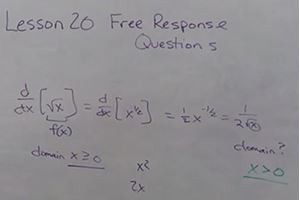Here we look at the overall structure of the AB exam and then focus the rest of the time on key free response question (FRQ) considerations. We have already rolled in many FRQs into the worksheet problems to begin preparing students. Typically solving these problems involves application of multiple concepts. It is important, in order to achieve a maximum score, to show all equations used and related work, along with proper units and explanations. We discuss several items to look out for. This lesson also discusses handling of absolute values. These can be removed by recognizing or establishing a constraint in the function's domain. Next we discuss how a domain can change after a mathematical operation. Ultimately, scoring well on the FRQs entails much more than just writing down a correct answer. Hopefully these insights and considerations will assist you in this endeavor.

#### 45 Lesson 20: Worksheet Review 14:29

This video goes through each of the practice problems from this lesson's worksheet. The answers are provided along with the methodology used to solve each problem, based on the lecture material. There is also a corresponding lesson answer key available, under the resource section, for quick reference.

#### 46 Closing Thoughts 03:30

In our final video we mainly provide an overview of the final exam and discuss preparations for taking the AP exam. The final exam structure is summarized in the final exam description. The key is to know your basic concepts and be well practiced in efficiently applying them to solve new problems. You should study, review lessons and worksheets, and do new practice problems and exams until you feel confident with the material. Key website URLs: https://apcentral.collegeboard.org/courses/ap-calculus-ab/exam (calculator requirements, sample problems and practice exams) https://apcentral.collegeboard.org/courses/ap-calculus-ab/exam/past-exam-questions (sample FRQ problems)

#### 47 Final Exam 00:00

As the actual AB exam is about 3 ½ hours long, it isn’t feasible to include all of the material into a single reasonable length final exam. However, between this final exam and the 20 worksheets that we have done together, we will have covered the full Calculus AB exam content. This overall final exam is limited to 1 hour and 23 minutes. For Part I, you have 20 min to solve 10 multiple choice questions without a calculator. For Part II, you have 18 min to solve 6 multiple choice questions with a calculator. Next, in Part III you have 15 minutes to solve a single multi-part free response question where a calculator is permissible. Lastly, for Part IV you have 30 min to solve two multi-part free response questions with a calculator. The time limit is given at the beginning of each section, as well as, whether a calculator can be used. No notes or textbook should be used.

Calculus AB Review Course

This is a comprehensive review course designed to prepare students for taking the AP Calculus AB exam through a single, intense, and concise course.  This was partly in consideration of those students with limited time that are juggling multiple AP and college credit classes.

It could be taken stand-alone, however, it would best serve as a supplement to go along with a high school Calculus AB, or similar course, to prepare for this AP exam. As it covers much of the material that you would find in a college Calculus I course, it could also be used as a supplement for this course.

Though this particular review course does not contain all of the AP Calculus BC exam material, it could still be a helpful supplement to prepare those students, as well.

Course Objective

Master the concepts and problem solving skills needed to score well on the AP Calculus AB exam.

Course Description

This Calculus AB review course is intended mainly as an comprehensive, but concise, review course for the AP Calculus AB exam. As such, lecture and practice problems are focused on the content of this AP exam. For instance, problems are structured to mimic how questions are asked and selected specifically to match expected test content to begin preparing the student for the exam. Furthermore, to enable an efficient review, time spent on specific concepts is weighed corresponding to observed exam focus (e.g., key pre-calculus topics).

Due to the extensive nature of the course, we necessarily cover a large range of topics. These are organized into logical and manageable sections and lessons. After covering key pre-calculus items, we cover topics including limits, continuity, Intermediate Value Theorem, derivatives, implicit differentiation, integrals, Riemann sums, area between curves, average value, integral substitution, particle motion, rates of change, related rates, volume of a solid, introduction to differential equations, and AP exam abstract and free response questions. All of this material is part of the scope of the AP Calculus AB exam.

There are other courses available here in both Calculus and pre-calculus, if students would like to delve deeper, extend upon the topics covered here, acquire additional practice, etc.

Course Content

• 20 lessons which are broken up into 44 videos
• about 7 hours of video
• lecture video for each lesson
• short worksheet to practice material from each lesson
• review video to go over answers and methodology for each worksheet problem
• answer key for each worksheet
• final exam with answer key

Suggested Audience

The course is strongly recommended for high school students preparing for the AP Calculus AB exam (typically 12th grade). It is also suggested as a course supplement for students taking high school Calculus AB / BC, college Calculus I, or equivalent courses. I say supplement for Calculus BC and college Calculus I, as this course only contains a subset of topics that you would find in those courses.

Furthermore, it would likely be helpful to those students that have taken or struggled with Calculus I, or an equivalent course, in the past and who thus need a comprehensive and efficient refresher on this material or are looking to better master the subject.

Requirements

Algebra I, Algebra II, Geometry, Pre-Calculus, and Calculus AB (or BC).

Algebra and trigonometry skills are a must. The course assumes prior mastery of Algebra, however, it does provide a limited pre-calculus section, including a lesson on key trigonometry concepts to assist students.

You will need a good graphing calculator. Here I used an older TI-83 Plus device in a few of the lessons to help students with crucial calculator functions needed for the AP exam. I've seen that newer models can provide some advantages, such as faster procesing time for solving integrals. Some of the worksheet problems require a graphing calculator. Please make sure to checkout what specific calculators are allowed by the exam before making a purchase. Also it is possible that your school or test location will have calculators available, however, again it would be prudent to inquire with them beforehand.

Credits

Teacher:  Mr. Copeland

Assistant: David

A special thanks to my son, David, for all his help in developing this course.

• Teacher: William
• Areas of expertise: Teaching 7-12 grade math, one-on-one and online tutoring of high school (AB and BC Calculus) and college Calculus (3 Levels, Multiple Universities)
• Education: Bachelor's and Master's of Science Degrees in Electrical Engineering
• Interests: Guitar, canoeing, strategy games
• Skills: Computer programming, photo analysis, algorithm development
• Associations: Michigan Technological University and Syracuse University Alumni
• Issues I care about: God, energy independence, space exploration

Mathematics can be challenging, but at the same time amazing. Once you spend time studying the material, it comes to life. As your understanding and skills grow you'll feel a sense of accomplishment. I had the privilege of applying advanced mathematics as a R&D contract engineer for the US Air Force for about 14 years.

#### Lesson 1 Worksheet Answer Key

Answer key for the worksheet for lesson 1

#### Lesson 2 Worksheet Answer Key

Answer key for the worksheet for lesson 2

#### Lesson 3 Worksheet Answer Key

Answer key for the worksheet for lesson 3

#### Lesson 4 Worksheet Answer Key

Answer key for the worksheet for lesson 4

#### Lesson 5 Worksheet Answer Key

Answer key for the worksheet for lesson 5

#### Lesson 6 Worksheet Answer Key

Answer key for the worksheet for lesson 6

#### Lesson 7 Worksheet Answer Key

Answer key for the worksheet for lesson 7

#### Lesson 8 Worksheet Answer Key

Answer key for the worksheet for lesson 8

#### Lesson 9 Worksheet Answer Key

Answer key for the worksheet for lesson 9

#### Lesson 10 Worksheet Answer Key

Answer key for the worksheet for lesson 10

#### Lesson 11 Worksheet Answer Key

Answer key for the worksheet for lesson 11

#### Lesson 12 Worksheet Answer Key

Answer key for the worksheet for lesson 12

#### Lesson 13 Worksheet Answer Key

Answer key for the worksheet for lesson 13

#### Lesson 14 Worksheet Answer Key

Answer key for the worksheet for lesson 14

#### Lesson 15 Worksheet Answer Key

Answer key for the worksheet for lesson 15

#### Lesson 16 Worksheet Answer Key

Answer key for the worksheet for lesson 16

#### Lesson 17 Worksheet Answer Key

Answer key for the worksheet for lesson 17

#### Lesson 18 Worksheet Answer Key

Answer key for the worksheet for lesson 18

#### Lesson 19 Worksheet Answer Key

Answer key for the worksheet for lesson 19

#### Lesson 20 Worksheet Answer Key

Answer key for the worksheet for lesson 20